American Journal of Civil Engineering
Volume 4, Issue 6, November 2016, Pages: 345-350

Nonlinear In-Plane Stability of Parabolic CFST Arch-Ribs with Cavity

Di Yun1, 2, Hao-wen Zheng1, 2, Ya-li Qi1, 3

1Department of Civil Engineering, Jilin Jianzhu University, Changchun, China

2Jilin Structure and Earthquake Resistance Technology Innovation Center, Changchun, China

3Department of Civil Engineering, Jilin Vocational Technical Engineering School, Siping, China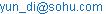(Di Yun)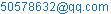(Hao-wen Zheng)

Di Yun, Hao-wen Zheng, Ya-li Qi. Nonlinear In-Plane Stability of Parabolic CFST Arch-Ribs with Cavity. American Journal of Civil Engineering. Vol. 4, No. 6, 2016, pp. 345-350. doi: 10.11648/j.ajce.20160406.21

Received: November 11, 2016; Accepted: November 30, 2016; Published: December 5, 2016

Abstract: Influences of cavity on in-plane stability behavior of parabolic CFST arch ribs are analyzed with ANSYS. Considering different cavity ratio, steel ratio and rise-to-span ratio, material and geometric nonlinearities analysis of parabolic CFST arch-ribs under different in-plane load cases are conducted. It is concluded that the bigger the cavity ratio is, the lower stiffness and bearing capacity of arch-ribs will be. With the same cavity ratio, including section cavity ratio and rib cavity ratio, there is more significant influence with the smaller steel ratio and the more uniform distribution of vertical loads. Moreover, there is little influence of common rise-to-span ratio on stability reduction due to the existence of cavity.

Keywords: Concrete Filled Steel Tube (CFST), Arch-Rib, Cavity, In-Plane Stability, Bearing Capacity

Contents

1. Introduction

It is very common in CFST bridges construction that there are cavities in the arch ribs. The cavity may be disengagement at the interface between the steel tube and the concrete, or voids in the core concrete. According to the reasons that resulted in the cavities, there are two main types of cavities [1,2,3]. The first type is radial or longitudinal disengagement essentially because of axial compression, temperature changing, concrete creep, failure of concrete expansion and the existence of separating board in steel tube. The other type is the voids in core concrete for unreasonable mixture ratio, quality problem in concrete pumping and etc. . It is obvious that the existence of the cavities breaks the efficient connection of the steel tube to the concrete thus influences the coincident between steel tube and concrete. Some rectifications should be made on current calculation theory and design methods which are based on the perfect co-working of steel and concrete.

After collecting the theory and design practice of the CFST arch bridges in many countries, which include the constitutive relationship, interaction, analysis and calculation of the ultimate bearing capacity theory, it is found that there is unified cognition but no accepted results about the cavity. Most researches on the cause of voids or cavities are qualitative analysis, researches on the influence of cavity on the arch-ribs’ stability are at beginning step [4,5]. In our country, there are four design codes for CFST bridges or members. Neither of them provides designing methods or formula for CFST arch-ribs with cavities . In summary, it is necessary to make sure the influences of the disengagement on the stiffness and bearing capacity of CFST arch ribs.

Focused on the cavity at the interface between steel tube and core concrete, the in-plane stability of parabolic CFST arch rib is analyzed with ANSYS. During the analysis, cavity ratio of composite section and cavity proportion of arch rib are mainly considered. The bearing capacity of the arch ribs under in-plane concentrated forces is obtained, including the influences of steel ratio, load cases, and rise-to-span ratio on stability behavior of the arch ribs with cavity.

It is found that the cavity nears the arch crown, which is between the left quarter-span and the right quarter-span, results in more apparent reduction on arch ribs’ in-plane stability. Meanwhile, the section cavity applied more significant reduction on elas-plastic stiffness than that on elastic stiffness. So more attention should be paid on ultimate bearing capacity compared to elastic bearing capacity in design.

2. Cavity Parameters

During the analysis, cavity condition is characterized by section cavity ratio ψ and rib cavity ratio ρ.

2.1. Section Cavity Ratio

Cavity ratio ψ is the ratio of cavity cross-section area to internal cross-section area of steel tube. This may thus be written as following: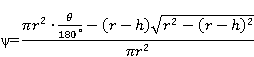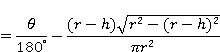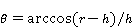(1)

where h = cavity height; r = internal radius of steel tube, as shown in Figure 1.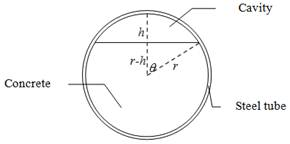Figure 1. Diagram of section cavity.

2.2. Rib Cavity Ratio

Rib cavity ratio ρ can be defined as the ratio of horizontal projection length of cavity part l’ to the rib span l as shown in Figure 2. That is ρ= l’/l. Along the rib span, cavity parts are symmetrical to the crown.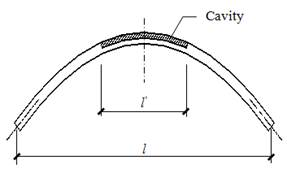Figure 2. Diagram of rib cavity.

The in-plane stability behavior of parabolic arch ribs with concrete filled single circular tube section is discussed by considering different ψ, ρ and steel ratio αs.

3. Finite Element Model

3.1. Geometrical Dimension

In reference 7, the experimental research on two parabolic CFST arch-ribs bearing in-plane single concentrated load was completed and the whole process from beginning to the ultimate status was obtained. Our FEM (Finite Element Modal) analysis is focused on the above parabolic arch ribs, that means the analysis model of this paper has the same geometrical dimensions of the experiment model in reference 7. The comparison among the analysis results of ANSYS, theory results and experiment results obtained in reference 7 was completed. It is concluded that the FEM results obtained with ANSYS are reasonable and believable .

During the analysis in this paper, the span of the arch-rib is 4.6m. The rise of arch rib is 1.5m, and then the rise-to-span ratio is 1/3. The arch axis equation is y=x2/3.45. The external diameter of steel tube is 76mm. The thickness of tube wall is 4mm.

3.2. Material Property

The stress-stain curve for steel tube is elastic-perfectly plastic material model based on GB50017-2003, which is the current steel structures design code of China. The most important characteristics of elastic-perfectly plastic steel model are its elastic modulus, Es = 2.06×105N/mm2 and its yield stress, fy = 310N/mm2. The stress-strain curve is the same in tension and compression, and the Poisson’s ratio is 0.283. In addition, the density of steel tube is 7850 kg/m3. Steel tube is simulated by using bilinear kinematic model BKIN in ANSYS.

Core concrete is simulated by using multi-linear isotropic model MISO in ANSYS. In according to current concrete structures design code, GB50010-2010, the stress-stain relationship for unreinforced concrete in a uniaxial stress state is taken as the equivalent stress-stain relationship for unrestrained concrete in a triaxial stress state. The property of concrete that is most quoted is its compressive strength fc=0.85fcu, where the normal cube strength fcu= 40N/mm2 obtained according to the concrete grade. In accordance with the equations suggested in Appendix C of GB50010-2010, the stress-stain curve for the core concrete of CFST arch rib is given in Figure 3. The maximum stress fc occurs at a strain of εc= 1.743×10-3=1743με after which the stress reduces. The plain concrete fails in crushing at a strain εu, and εu= 3.643×10-3=3643με. The accent coefficient αa and decent coefficient αd are 1.848 and 1.756 respectively .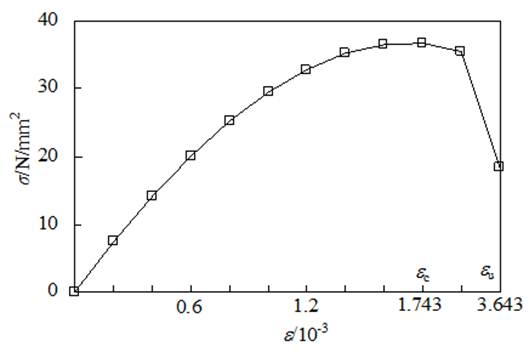Figure 3. Stress-strain curve of core concrete.

3.3. Modeling

The composite section, including circular tube and core concrete, is modeled by custom section function of BEAM189 in ANSYS. Both the external tube and the core concrete can be simulated by PLANE82 with respective geometrical dimensions and material properties. In order to ensure the deformation compatibility at the interface between the steel and concrete components, the mesh dimensions of finite element model (FEM) are determined carefully. Because of the existence of common nodes, there is no sliding between the steel tube and core concrete so the plane-section assumption is satisfied.

It is worth noting that the arch ends are fixed during the analysis in this paper.

4. Results of FEM Analysis

At first, the model of a single arch rib without section cavity is built. Vertical concentrated load are applied at the arch crown and quarter-span respectively. A combination of material and geometric nonlinearities is considered during FEM analysis. Load-vertical displacement (P-uy) curves are shown in Figure 4 & 5. It is worth noting that the negative displacement value in Figure 4 & 5 means the displacement is in the opposite direction to external force.

The deformation diagrams are shown in Figure 6. It can be seen that the deformation plot is symmetric when the force is at crown and the deformation plot is anti-symmetric when the force is at quarter-span section. Correspondingly, the capacity of the arch rib under symmetric load case is 47.5kN, which is bigger than that one beard quarter-span load, 33.6kN.

4.1. Influence of Section Cavity Ratio

When the cavity height h are 0, 7mm and 17mm respectively, section cavity ψ are 0, 10% and 20% correspondingly according to the formula 1. The in-plane stability behavior of CFST arch ribs with the above different ψ is analyzed, utilizing arch-length method and considering geometric and material nonlinearities. When conducting the above analysis, the arch ribs are applied vertical concentrated load at the crown and quarter-span respectively. Although with different section cavity ratio, the buckling modes are very similar to that ones shown in Figure 6.Figure 4. Pu-uy curves when load applied at crown.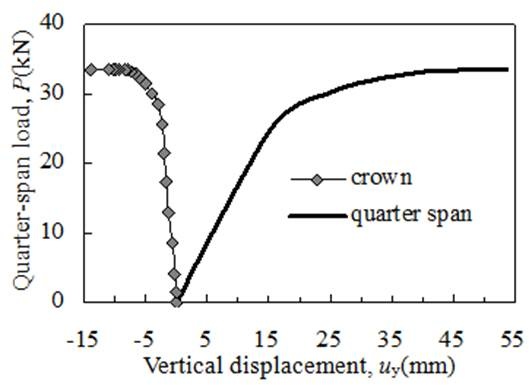Figure 5. Pu-uy curves when load applied at quarter-span.

It can be concluded that in-plane buckling mode does not change with the change in section cavity ratio.As shown in Figure 7 & 8, load-displacement curves are almost coincident in the elastic range. It can be concluded that there is little influence on rib’s elastic stiffness due to increasing section cavity ratio ψ. Unlike elastic stiffness of arch ribs, the in-plane stability capacity decreases as section cavity ratio ψ increases because of the consideration of nonlinearities. When ψ is 20%, the in-plane capacity is 6.78% lower than that one of ψ= 0.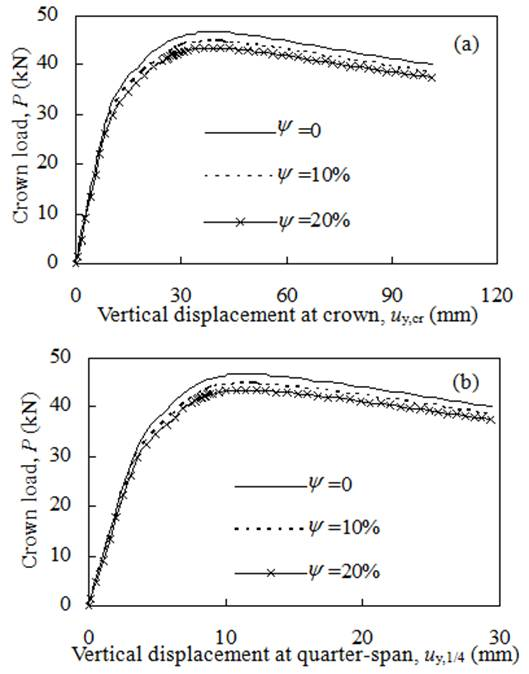Figure 7. Influence of section cavity ratio ψ on crown load-displacement curves.

It is worth noting that though different load position causes different deformation, the variety trend and degree of in-plane capacity and stiffness of the arch ribs with different section cavity ratio are very similar. In view of limit space, crown loading is mainly discussed when the analysis is focused on single-point force hereafter.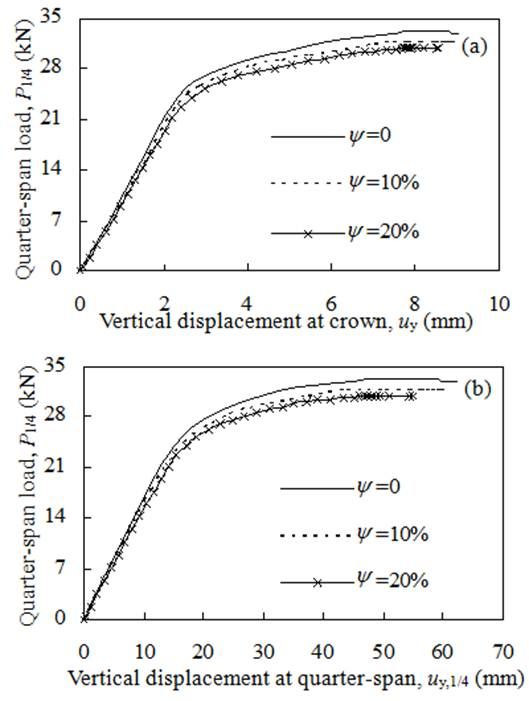Figure 8. Influence of section cavity ratio ψ on quarter-span load-displacement curves.

4.2. Influence of Steel Ratio αs

Steel ratio may be found in a number of books for composite members design. It is always convenient to express the ratio in terms of As/Ac, where As is the cross-section area of steel tube and Ac is the cross-section area of core concrete. The in-plane capacity of arch-rib with different section cavity ratio ψ and steel ratio αs is calculated. The in-plane vertical load is applied to the arch rib at the crown and the arch ends are all fully fixed. When steel ratio αs changes from 20% to 10%, the arch-rib’s stiffness and capacity fall sharply as shown in Figure 9.

Furthermore, as the steel ratio decreases, the influence of cavity on arch rib’s in-plane capacity is more apparent. When αs=20%, the bearing capacity of the arch rib without cavity (ψ=0) is 7.36% higher than that one of ψ=20%; When αs=10%, the bearing capacity of the arch rib without cavity (ψ=0) is 15.63% higher than that one of ψ=20%.

This is principally because that the steel tube makes a bigger contribution to the rib’s stiffness. When αs is decreased, the stiffness reduction due to cavity in core concrete becomes worse. The steel ratio αs of CFST arch bridges in practice is usually between 4.5% and 10% , it is necessary to pay more attention to the cavity problem of arch ribs.

4.3. Influence of Rib Cavity Ratio ρ

When αs=10% and ψ=20%, the in-plane bearing capacity Pcr,in of arch ribs with different ρ is obtained. As rib cavity ratio ρ increases, the cavity part along the rib span becomes longer. It is found that Pcr,in decreases as ρ increases as shown in Figure 10.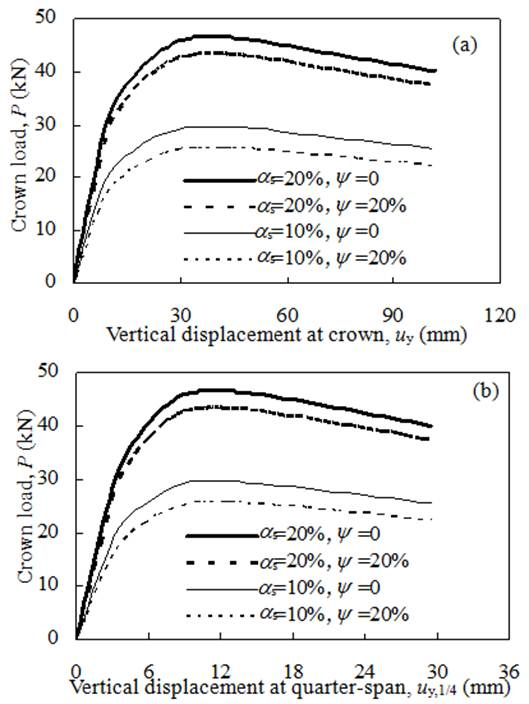Figure 9. Influence of steel ratio αs on crown load-displacement curves

When ρ is less than 1/2, Pcr,in-ρ curve slides more quickly (Fig.10); When ρ=1/2, Pcr,in is 10.45% lower than that one of the arch rib without cavity. After that, Pcr,in falls slowly as ρ increases continually. Pcr,in falls by 13.51% when ρ=1,which means section cavity is all through the span.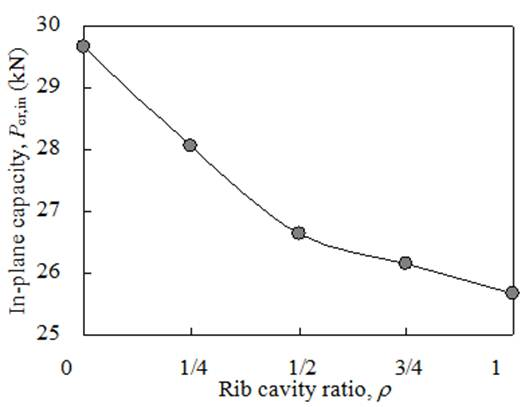Figure 10. Influence of rib cavity ratio ρ on in-plane capacity Pcr,in-ρ.

The change of falling speed of in-plane capacity mainly depends on the distribution of section axial force of arch rib and the distance between the ends of cavity part to arch ends. From the quarter-span to arch crown, section axial force varies from maximum to minimum, and then decreases slowly from quarter-span to arch end. Moreover, the farther distance from the cavity section to the arch ends, the weaker the constraint is.

In practice, bridge deck load transfers evenly to arch ribs by suspenders and pillars. Thus arch ribs work under distributed concentrated forces instead of single-point concentrated force. It is necessary to discuss the in-plane capacity Pcr,in of the arch rib under multi-point vertical concentrated force. Nonlinear analysis of arch ribs under distributed concentrated forces is completed when αs=10%,ψ=20% and ρ are 0,1/2 and 1 respectively. The load cases includes single concentrated force at arch crown, three distributed concentrated forces at each quarter span and seven distributed concentrated forces at every 1/8 span. At last, the comparison among analysis results of the above load cases is conducted in order to make sure the influence of different load cases on the arch rib’s in-plane bearing capacity, Pcr,in.

The relationship curves between total load ΣP and the vertical displacement at crown uy are presented in Figure 11. Here, the ΣP is the sum of all distributed forces being acted on the arch-rib, which equals to the number of forces times the value of each vertical concentrated force.

Compared to the single-point concentrated load case, the in-plane deformation of the arch rib at each load applied point suffers more restriction as the number of distributed forces increases. Thus it can be seen in Figure 11 that the ascent stage of ΣP-uy curves becomes more steeply as the number of distributed concentrated forces increasing, which means the arch rib under distributed concentrated forces has bigger in-plane stiffness. As a result, the more distributed forces applied on the arch rib, the higher Pcr,in the arch rib gets as shown in Figure 11.

On the other side, as the number of distributed concentrated forces increases, the plastic deformation ability of arch ribs decreases apparently. The ΣP-uy curves fall more disastrously as the number of concentrated forces increases as shown in Figure 11.

4.5. Influence of Rise-to-Span Ratio

Based on the stability theory of arch structures, better stability can be obtained when rise-to-span ratio f/l is near 1/4 . In view of the common value of f/l in practice , Pcr,in is calculated while f/l are 1/3, 1/4 and 1/5 respectively with αs =10%, ψ=20%. The rib cavity ratio ρ are 0 and 1 respectively. In addition, load case is single-point concentrated force during the above calculation. Pcr,in-f/l curves are nearly parallel as shown in Figure 12. It is found that there is no interplay between cavity and rise-to-span ratio. Thus there is no influence of f/l on stability reduction due to the existence of cavity.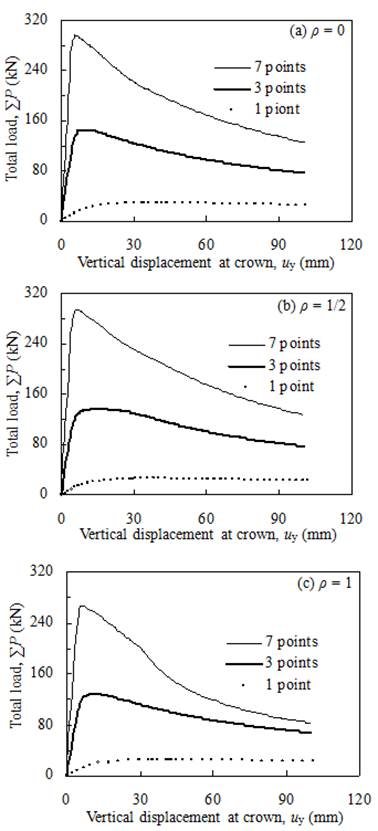Figure 11. Influence of load cases on ΣP-uy curves.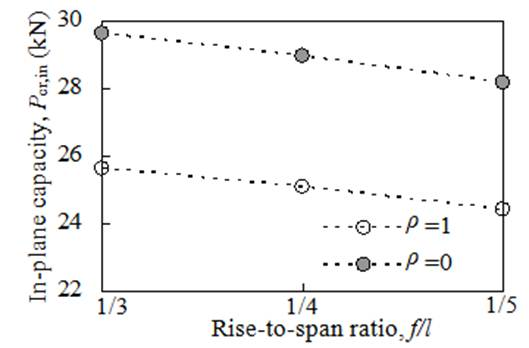Figure 12. Influence of f/l on Pcr,in of arch ribs with cavity.

5. Conclusion

There is little influence of section cavity on elastic stiffness of the arch rib. But after elastic stage, the arch rib stiffness decreases apparently as section cavity ratio increasing. Meanwhile, in-plane bearing capacity Pcr,in of the arch rib decreases with increasing cavity ratio.

The smaller the steel ratio is, the more significant influence of cavity on stability of the arch rib. Thus the lower Pcr,in will be obtained. Because common steel ratio in practice CFST arch bridges is usually less than 10%, it is necessary to pay more attention to the cavity problem of CFST arch ribs.

When the cavity is between left quarter-span to right quarter-span, the influence of rib cavity on in-plane bearing capacity is more significant.

Compared to single concentrated force applied on arch crown, the load case of multi-point distributed concentrated forces produced larger stiffness and higher in-plane bearing capacity. It is worth noting that distributed concentrated forces reduce the plasticity deformation of arch ribs.

There is no influence of common rise-to-span f/l on stability reduction due to the existence of cavity.

Acknowledgements

This research work is supported by the National Natural Science Foundation of China (Grant No. 51308254).

References

1. C. J. Lin, J. L. Zheng, and R. Qin, "Research review on incompletion filling of CFST members,"China: Journal of China and Foreign Highway. vol. 24 (6),2004,pp. 54–58.
2. K. F. Liang, "Influence of separation on mechanic behavior of CFST members," Changsha: Hunan University, 2008, pp. 1-12.
3. J. K. Xin,"Classification and reasons of separation of CFST arch ribs," China: China Science and Technology Information. vol. 23 (12)2009, pp.81–82.
4. J. C. Su, "Survey on CFST arch bridges and research on their void problem," Chengdu: Southwest Jiaotong University, 2012, pp: 1-23, 76-85.
5. S. Wang, "Effect of separation on the static and dynamic performance of concrete-filled steel tube arch bridge," Xi’an: Chang’an University, 2012, pp: 1-10, 34-44.
6. H. W. Zheng, D. Yun, "Calculation methods for the in-plane stability of concrete filled steel tubular arch ribs," China: Steel Construction. vol. 31(10) 2016, pp: 71-75.
7. B. C. Chen, Y. J. Chen, "Experimental research on the whole process of CFST arch rib under in-plane load case," China: Engineering Mechanics. vol. 17 (2) 2000, pp. 44-50.
8. D. Yun, H. W. Zheng and H. Zhou, "Influence of filling-incompletion on in-plane bearing capacity of single concrete filled steel tubular arch ribs," China: Journal of Building Structures. vol. 36 (sup.1) 2015, pp: 120-125.
9. China Association for Engineering Construction Standardization, "Code for design of steel structures, GB50017-2003," Beijing: China Building Industry Press, 2003.
10. Housing and Urban-rural Development of the People’s Republic of China, "Code for design of concrete structures, GB50010-2010," Beijing: China Building Industry Press, 2010.
11. Z. Q. Zou, S. Q. Song, "Application of multiaxial strength and constitutive relation of concrete," Shanxi: Shanxi Structures. vol. 152 (2), 2008, pp. 13-17.
12. B. C. Chen, F. ZH.Liu, J. G. Wei, "Statistic and analysis of 327 CFST arch bridges," China: Journal of China and Foreign Highway. vol.31(3),2011,pp.96103.
13. H. F. Xiang, G. D. Liu, "Stability and vibration of arch structures," Beijing: China Communications Press. 1991, pp. 129-157.

 Contents 1. 2. 2.1. 2.2. 3. 3.1. 3.2. 3.3. 4. 4.1. 4.2. 4.3. 4.4. 4.5. 5.
Article ToolsAbstractPDF(446K)